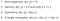# K Nearest Neighbors

KNN is a supervised algorithm which is based on assumption that similar classes are grouped together. KNN is a non parametric technique since it does not basis any assumptions about the distribution of underlying data. When we say they are closer, this proximity is seen by some mathematical notation like distance.

KNN Algorithm can be summarized as below

1.Load the data and standardize feature variables prior to running KNN

2. Initialize K to your chosen number of neighbors

3. For each observation of the data calculate the distance between that observation and every other observation from the data.

4. Sort the ordered collection of distances in ascending order by the distances

5. Pick the first K entries from the sorted collection

6. Get the class labels from that K entries

7. If regression, return the mean or median of the K labels

8. If classification, return the mode of the K labels or alternatively class label with the highest probability value

where I is the Indicator variable if class label is j(1) or not(0)

Now major doubt is

How to select appropriate K:

• Please Note that the value of K controls the Bias — variance Tradeoff in this case. So by reducing the value of K to 1, we are overfitting and hence our bias reduces, variance increases. However if we increase the value of K to a very large value, we are increasing our bias, by reducing the variance.
• We can use Cross Validation to choose K that achieves minimum hold-out error. We specifically can use V fold which is based on multiple data splitting. More precisely, the data are divided in V (non overlapping) sets. Each set is hold-out and used to compute an hold-out error which is eventually averaged to obtained the final V -fold cross validation error.

Read more about Cross Validation here

How to chose distance formula

For this one thing is to create vector of each observation which is nothing but the n tuple based on Xi variables like (X1, X2, …Xn). There are many metrics that can be used(following certain properties as below)d stands for distance metric used
• Minkowski
• Manhattan(p=1 in Minkowski)
• Euclidean(p=2 in Minkowski)

Most common distance measure

• Cosine

Visualize this as a dot product, closer this value to 1, more similar the two vectors are.

• Hamming

Check for dissimilar letters in a word index by index.

# Advantages

1. The algorithm is simple and easy to implement.
2. There’s no need to build a model, tune several parameters, or make additional assumptions.
3. The algorithm is versatile. It can be used for classification, regression, and search (as we will see in the next section).

# Disadvantages

1. The algorithm gets significantly slower as the number of examples and/or predictors/independent variables increase.

Now that we know the basic intuition of KNN is, we jump onto understanding what Weighted KNN is right here in this post.

Resources:

Problem Solver, Data Science, Actuarial Science, Knowledge Sharer, Hardcore Googler

## More from Shaily jain

Problem Solver, Data Science, Actuarial Science, Knowledge Sharer, Hardcore Googler